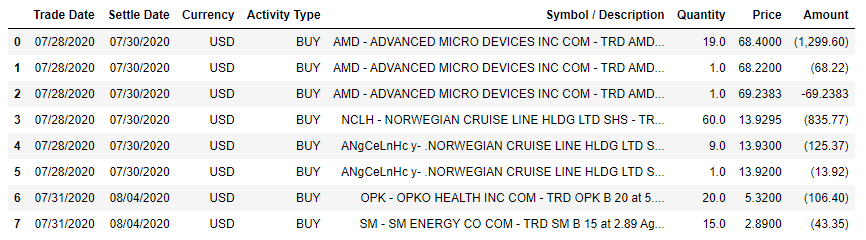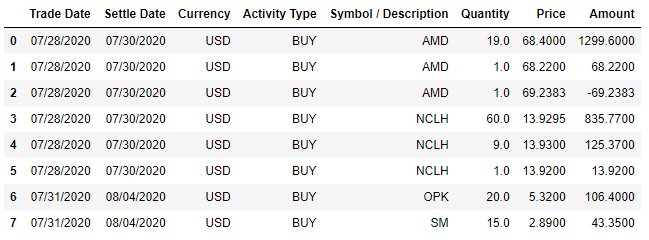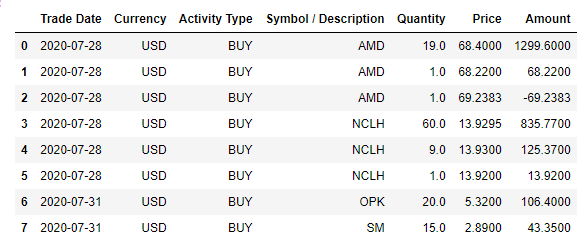Search

# Automate Revolut trading tax calculation with Pandas

Updated: Apr 5

Last year I discovered how easy it can be to trade with the Revolut app. I was playing for a couple of months, in the beginning, I did few transactions but then I was curious if I can make a profit from a higher number of transactions, a kind of day trading. Short story, I realized that day trading is not for me. Now, in 2021, I had to calculate my realized capital gains from 2020 trading to report for tax.

If you have few trading transactions then is really easy to compute the realized capital gains, you just need to subtract from the selling price the buying price, for example:

1. you buy 10 MSFT shares for 1800\$ (180\$ for each share)

2. you are selling all of them for 2200 (220\$ each)

3. to compute the realized capital gain: 2200\$ - 1800\$ = 400\$

But if you bought n1 shares then you sold k1 (k1 < n1) then you bought another n2, and then you sold k2 (k2 < n2 + n1 - k1) shares and so on it becomes a bit hard to take track of all of them and to compute the realized capital gains. Here is an example:

1. you buy 2 MSFT shares for 360\$(180\$ each)

2. you buy 5 MSFT shares for 1000\$( 200\$ each), at this point, you have 7 MSFT (~194.286\$ each, you have to use the weighted average)

3. you are selling 4 for 796\$ (199\$ each), you still have 3 more shares (~194.286\$ each), you have a capital gain of (199\$ - 194.286\$) * 4 = 18.856 \$

4. you are buying 10 shares for 2200\$ (220\$ for each), you have 13 shares (~214.07\$ each)

5. you are selling 5 for 1150\$(230\$ each), you have 8 shares (~214.07\$ each), and a total realized capital gain of (230\$ - 214.07\$) * 5 + 18.856\$ ~= 98.51\$

After this example imagine that you do not have only one stock but 20-30 and for each stock, you did form 2 to 20 transactions. It will take a lot of time if you want to compute the realized capital gains by hand or even in Excel, only to get the data by hand and to double-check yourself that you did it correctly it may take hours if not an entire day. This was my scenario, so I decided to use a Jupyter notebook and Pandas in order to automate Revolut trading tax calculation.This picture perfectly expresses my feelings after I calculated my Revolut trading tax with Pandas in less than 5 minutes (obviously, after I did spend a few hours writing and testing my code). If you want to become a Kung Fu Panda tax warrior you must follow this post until the end.

## From where do you take your data (the transaction history)?

In order to get your transaction history, you have to download your trading account statements. Link here if you do not know where to look.

Once you downloaded all your statements you have to convert them from pdf to CSV/excel, for simplicity you can do it online if you do not care too much about your data or you can do it using tabula-py :

`!pip install tabula-py`

Here is an example of how to convert one statement pdf to CSV:

```import tabula

df = r"C:\Users\dell\Downloads\August.pdf"
output = r"C:\Users\dell\Downloads\converted_august.csv"
tabula.convert_into(df, output, output_format="csv", stream=True, pages='all')```

## Getting the right table

The account statements have more than one type of table, the ones that we want are those that have the "Activity Type" column, the values in these columns are "BUY" and "SELL" (for most of the rows).

To get the tables in padas we will use the "pandas.read_clipboard" method, first you have to select the entire area of the right table and copy:

```import pandas as pd

# assuming that you started to trade in July
df_july = pd.read_clipboard()
df_july```The used data looks like real data but is fake data.

## Data cleaning

### Keep only the activity type BUY or SELL

```activities = {'BUY', 'SELL'}
df_july = df_july[df_july['Activity Type'].isin(activities)].reset_index(drop=True)
df_july```### Use only symbols

```symbols = {'AMD', 'NCLH', 'OPK', "SM"}
df_july['Symbol / Description'] = [next(s for s in symbols if s in e) for e in df_july['Symbol / Description']]
df_july```### Change column Amount data type to float

`df_july.dtypes`
```Trade Date               object
Settle Date              object
Currency                 object
Activity Type            object
Symbol / Description     object
Quantity                float64
Price                   float64
Amount                   object
dtype: object```

```import re
df_july.Amount = [float(re.sub('[,()]', '', n)) for n in df_july.Amount]
df_july```### Remove the Settle Date column

```df_july.drop('Settle Date', axis=1, inplace=True) # where 1 is the axis number (0 for rows and 1 for columns)
df_july

```### Change the Trade Date column data type to datetime

```df_july['Trade Date']= pd.to_datetime(df_july['Trade Date'])
df_july```### Rename column Symbol / Description into Symbol

```df_july.rename(columns={'Symbol / Description': 'Symbol'}, inplace=True)
df_july```## Monthly data stats

For each row from each table, we want to compute the average price, keep track of the current "Total amount" at each step, and store in a list the profit or the loss together with the trading day after each sale. The profit or the loss is in \$ so to compute the trading tax we have to convert in the local currency, the exchange rate has to be the average exchange rate from the day you had the profit or the loss. This may depend on your country but this rule is applying in my country Romania.

```from collections import defaultdict

monthly_data = defaultdict(lambda: defaultdict(lambda: defaultdict(int)))```

Do not get "scare" about this variable, this is how data stored by this variable will look:

```defaultdict(<function __main__.<lambda>()>,
'August': defaultdict(<function __main__.<lambda>.<locals>.<lambda>()>,
{'AMD': defaultdict(int,
{'Avg Price': 68.87360322580645,
'Total Quantity': 31.0}),
'NCLH': defaultdict(int,
{'Avg Price': 13.92942857142857,
'Total Quantity': 70.0}),
'OPK': defaultdict(int,
{'Avg Price': 4.9216037735849065,
'Total Quantity': 530.0}),
'SM': defaultdict(int,
{'Avg Price': 2.89,
'Total Quantity': 15.0}),
'FSLY': defaultdict(int,
{'Avg Price': 0,
'Total Quantity': 0.0,
'Profit': [(Timestamp('2020-08-07 00:00:00'),
360.22)]}),
'NKLA': defaultdict(int,
{'Avg Price': 0,
'Total Quantity': 0.0,
'Profit': [(Timestamp('2020-08-07 00:00:00'),
-4.23)]})})```

To compute the weighted average at each step (when we make a buy action) we will need a function:

```def compute_avg_price_total_amount_quantity(symbol, quantity, symbol_data, current_amount):

total_amount = symbol_data['Avg Price'] * symbol_data['Total Quantity'] + current_amount
total_quantity = symbol_data['Total Quantity'] + quantity
symbol_data['Avg Price'] = total_amount / total_quantity
symbol_data['Total Quantity'] = total_quantity ```

To compute the profit/loss at each sell action we will need another function:

```def compute_profit_update(symbol, quantity, symbol_data, data_time, current_amount):

assert abs(quantity) <= symbol_data['Total Quantity']  , f"{symbol}, {data_time}, quantity = {abs(quantity)}, Total Quantity = {symbol_data['Total Quantity']}"
profit = round(current_amount + symbol_data['Avg Price'] * quantity,5)
symbol_data['Total Quantity'] = symbol_data['Total Quantity'] + quantity

if symbol_data['Total Quantity'] == 0:
symbol_data['Avg Price'] = 0

if isinstance(symbol_data['Profit'], int):
symbol_data['Profit'] = [(data_time, profit)]
else:
symbol_data['Profit'].append((data_time, profit))```

Now we are ready to compute the monthly stats for July: